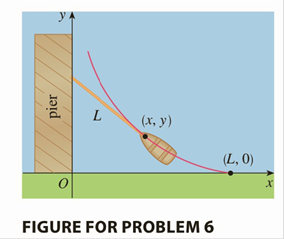Chapter 7.P, Problem 6P

Chapter
Section
Textbook Problem
1 views

# A man initially standing at the point O walks along a pier pulling a rowboat by a rope of length L. The man keeps the rope straight and taut. The path followed by the boat is a curve called a tractrix and it has the property that the rope is always tangent to the curve (see the figure).(a) Show that if the path followed by the boat is the graph of the function y = f ( x ) , then f ′ ( x ) = d y d x = − L 2 − x 2 x (b) Determine the function y = f ( x ) .To determine

( a)

To show:

That if the path followed by the boat is y=f(x), then f(x)=dydx=L2x2x.

Explanation

Given:

y=f(x)

Formulae used:

The differentiation method

Consider y=f(x)

Hence, use the differentiation method

Now according to the given expression, the value of the intersection of the given expression y=f(x) is

As given in the question a triangle will form

Therefore,

tanθ=L2x2x

But slope,

dydx=tanθ=L<

To determine

(b)

The function y=f(x).

### Still sussing out bartleby?

Check out a sample textbook solution.

See a sample solution

#### The Solution to Your Study Problems

Bartleby provides explanations to thousands of textbook problems written by our experts, many with advanced degrees!

Get Started

#### let f(x) = x3 + 5, g(x) = x2 2, and h(x)= 2x + 4. Find the rule for each function. 2. f g

Applied Calculus for the Managerial, Life, and Social Sciences: A Brief Approach

#### True or False: If , then converge absolutely.

Study Guide for Stewart's Multivariable Calculus, 8th

#### What rationalizing substitution should be made for 1x+x5dx? a) u = x b) u=x c) u=x5 d) u=x10

Study Guide for Stewart's Single Variable Calculus: Early Transcendentals, 8th